## Binary Tree-

Before you go through this article, make sure that you gone through the previous article on Binary Trees.

We have discussed-

• Binary tree is a special tree data structure.
• In a binary tree, each node can have at most 2 children.

## Tree Traversal-

 Tree Traversal refers to the process of visiting each node in a tree data structure exactly once.

Various tree traversal techniques are-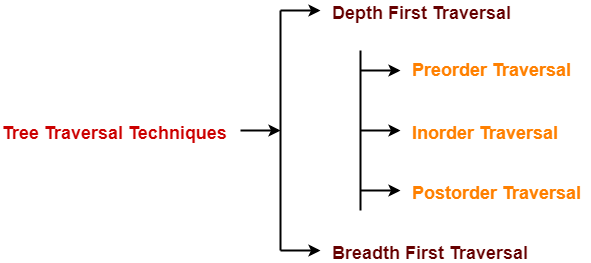## Depth First Traversal-

Following three traversal techniques fall under Depth First Traversal-

1. Preorder Traversal
2. Inorder Traversal
3. Postorder Traversal

## Algorithm-

1. Visit the root
2. Traverse the left sub tree i.e. call Preorder (left sub tree)
3. Traverse the right sub tree i.e. call Preorder (right sub tree)

Root  Left  Right

## Example-

Consider the following example-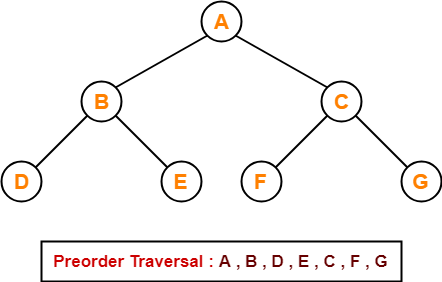## Preorder Traversal Shortcut

Traverse the entire tree starting from the root node keeping yourself to the left.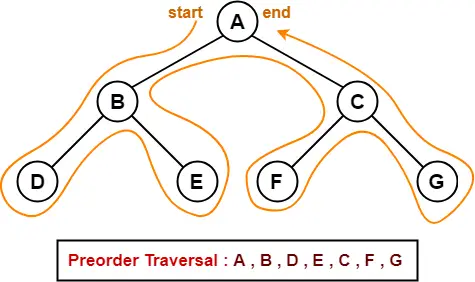## Applications-

• Preorder traversal is used to get prefix expression of an expression tree.
• Preorder traversal is used to create a copy of the tree.

## Algorithm-

1. Traverse the left sub tree i.e. call Inorder (left sub tree)
2. Visit the root
3. Traverse the right sub tree i.e. call Inorder (right sub tree)

Left  Root  Right

## Example-

Consider the following example-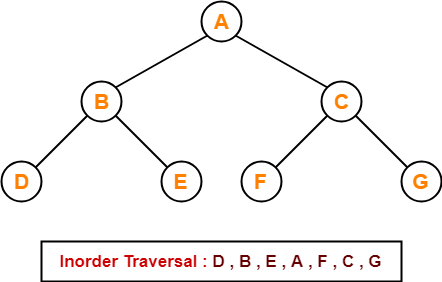## Inorder Traversal Shortcut

Keep a plane mirror horizontally at the bottom of the tree and take the projection of all the nodes.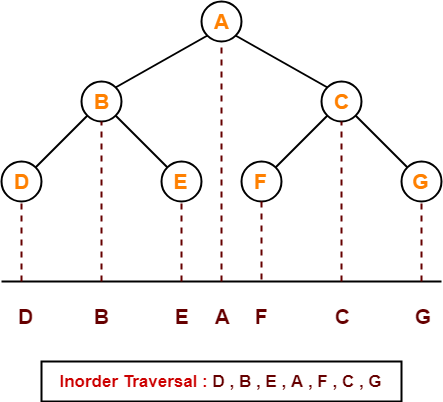## Application-

• Inorder traversal is used to get infix expression of an expression tree.

## Algorithm-

1. Traverse the left sub tree i.e. call Postorder (left sub tree)
2. Traverse the right sub tree i.e. call Postorder (right sub tree)
3. Visit the root

Left  Right  Root

## Example-

Consider the following example-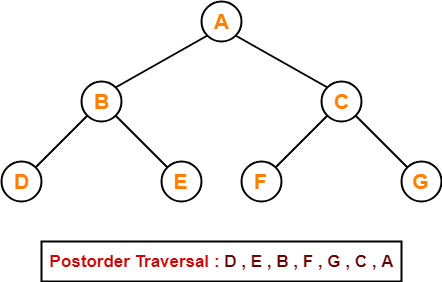## Postorder Traversal Shortcut

Pluck all the leftmost leaf nodes one by one.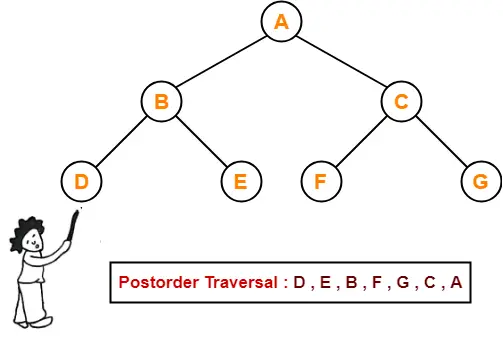## Applications-

• Postorder traversal is used to get postfix expression of an expression tree.
• Postorder traversal is used to delete the tree.
• This is because it deletes the children first and then it deletes the parent.

• Breadth First Traversal of a tree prints all the nodes of a tree level by level.
• Breadth First Traversal is also called as Level Order Traversal.

## Example-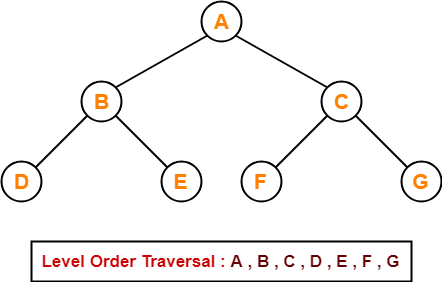## Application-

• Level order traversal is used to print the data in the same order as stored in the array representation of a complete binary tree.

To gain better understanding about Tree Traversal,

Watch this Video Lecture

## Problem-01:

If the binary tree in figure is traversed in inorder, then the order in which the nodes will be visited is ____?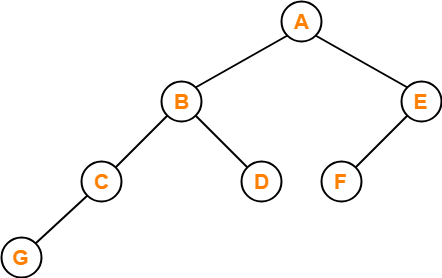## Solution-

The inorder traversal will be performed as-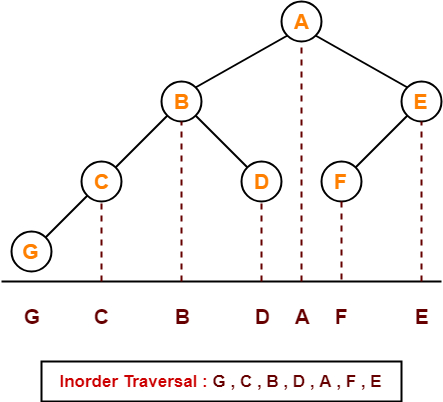## Problem-02:

Which of the following sequences denotes the postorder traversal sequence of the tree shown in figure?1. FEGCBDBA
2. GCBDAFE
3. GCDBFEA
4. FDEGCBA

## Solution-

Perform the postorder traversal by plucking all the leftmost leaf nodes one by one.

Then,

Postorder Traversal :  G , C , D , B , F , E , A

Thus, Option (C) is correct.

## Problem-03:

Let LASTPOST, LASTIN, LASTPRE denote the last vertex visited in a postorder, inorder and preorder traversal respectively of a complete binary tree. Which of the following is always true?

1. LASTIN = LASTPOST
2. LASTIN = LASTPRE
3. LASTPRE = LASTPOST
4. None of these

## Solution-

Consider the following complete binary tree-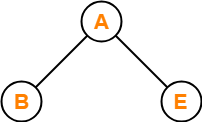Preorder Traversal  : B , A , E

Inorder Traversal     : B , A , E

Postorder Traversal : B , E , A

Clearly, LASTIN = LASTPRE.

Thus, Option (B) is correct.

## Problem-04:

Which of the following binary trees has its inorder and preorder traversals as BCAD and ABCD respectively-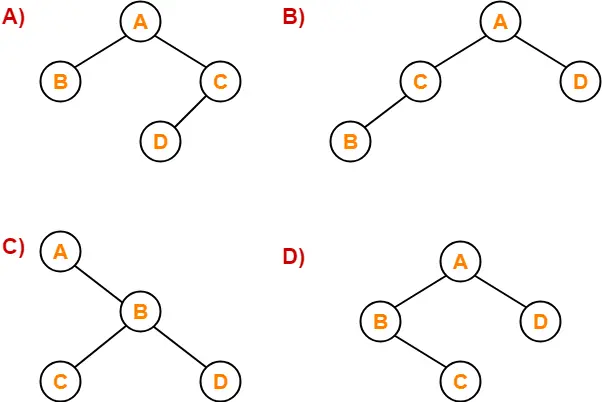## Solution-

Option (D) is correct.

To watch video solutions and practice more problems,

Watch this Video LectureNext Article- Binary Search Trees

Get more notes and other study material of Data Structures.

Watch video lectures by visiting our YouTube channel LearnVidFun.

## Binary Tree-

Before you go through this article, make sure that you gone through the previous article on Binary Trees.

We have discussed-

• Binary tree is a special tree data structure.
• In a binary tree, each node can have at most 2 children.
• There are following types of binary trees-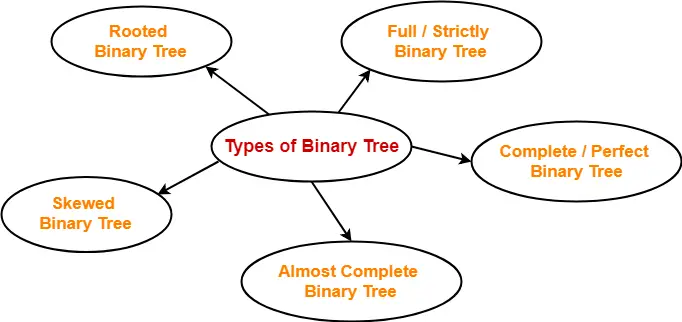## Binary Tree Properties-

Important properties of binary trees are-

## Property-01:

 Minimum number of nodes in a binary tree of height H= H + 1

### Example-

To construct a binary tree of height = 4, we need at least 4 + 1 = 5 nodes.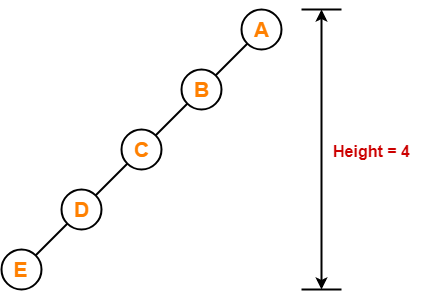## Property-02:

 Maximum number of nodes in a binary tree of height H= 2H+1 – 1

### Example-

Maximum number of nodes in a binary tree of height 3

= 23+1 – 1

= 16 – 1

= 15 nodes

Thus, in a binary tree of height = 3, maximum number of nodes that can be inserted = 15.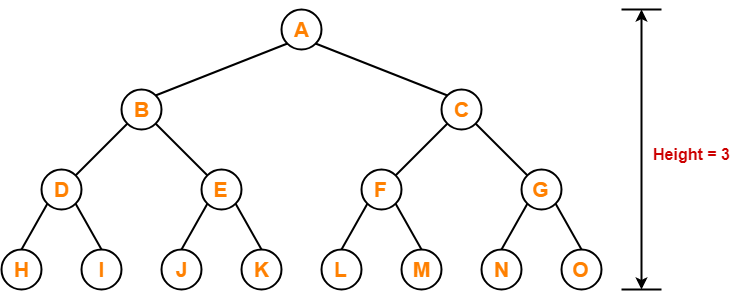We can not insert more number of nodes in this binary tree.

## Property-03:

 Total Number of leaf nodes in a Binary Tree= Total Number of nodes with 2 children + 1

### Example-

Consider the following binary tree-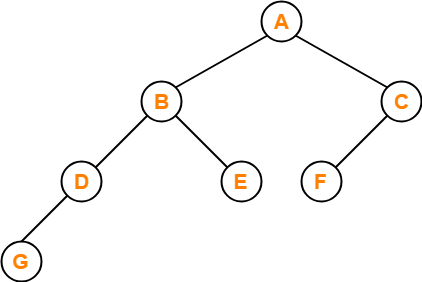Here,

• Number of leaf nodes = 3
• Number of nodes with 2 children = 2

Clearly, number of leaf nodes is one greater than number of nodes with 2 children.

This verifies the above relation.

## NOTE

It is interesting to note that-

Number of leaf nodes in any binary tree depends only on the number of nodes with 2 children.

## Property-04:

 Maximum number of nodes at any level ‘L’ in a binary tree= 2L

### Example-

Maximum number of nodes at level-2 in a binary tree

= 22

= 4

Thus, in a binary tree, maximum number of nodes that can be present at level-2 = 4.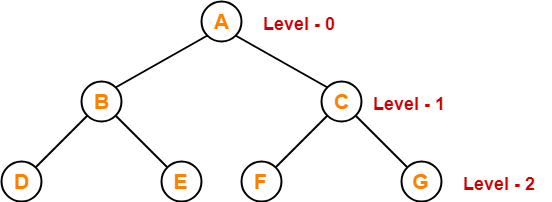To gain better understanding about Binary Tree Properties,

Watch this Video Lecture

## Problem-01:

A binary tree T has n leaf nodes. The number of nodes of degree-2 in T is ______?

1. log2n
2. n-1
3. n
4. 2n

## Solution-

Using property-3, we have-

Number of degree-2 nodes

= Number of leaf nodes – 1

= n – 1

Thus, Option (B) is correct.

## Problem-02:

In a binary tree, for every node the difference between the number of nodes in the left and right subtrees is at most 2. If the height of the tree is h > 0, then the minimum number of nodes in the tree is ______?

1. 2h-1
2. 2h-1 + 1
3. 2h – 1
4. 2h

## Solution-

Let us assume any random value of h. Let h = 3.

Then the given options reduce to-

1. 4
2. 5
3. 7
4. 8

Now, consider the following binary tree with height h = 3-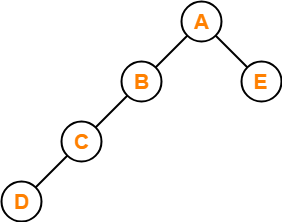• This binary tree satisfies the question constraints.
• It is constructed using minimum number of nodes.

Thus, Option (B) is correct.

## Problem-03:

In a binary tree, the number of internal nodes of degree-1 is 5 and the number of internal nodes of degree-2 is 10. The number of leaf nodes in the binary tree is ______?

1. 10
2. 11
3. 12
4. 15

## Solution-

Using property-3, we have-

Number of leaf nodes in a binary tree

= Number of degree-2 nodes + 1

= 10 + 1

= 11

Thus, Option (B) is correct.

## Problem-04:

The height of a binary tree is the maximum number of edges in any root to leaf path. The maximum number of nodes in a binary tree of height h is ______?

1. 2h
2. 2h-1 – 1
3. 2h+1 – 1
4. 2h+1

## Solution-

Using property-2, Option (C) is correct.

## Problem-05:

A binary tree T has 20 leaves. The number of nodes in T having 2 children is ______?

## Solution-

Using property-3, correct answer is 19.

To watch video solutions and practice more problems,

Watch this Video LectureNext Article- Binary Tree Traversal

Get more notes and other study material of Data Structures.

Watch video lectures by visiting our YouTube channel LearnVidFun.

## Tree Data Structure-

Before you go through this article, make sure that you have gone through the previous article on Tree Data Structure.

We have discussed-

• Tree is a non-linear data structure.
• In a tree data structure, a node can have any number of child nodes.

## Binary Tree-

 Binary tree is a special tree data structure in which each node can have at most 2 children.Thus, in a binary tree,Each node has either 0 child or 1 child or 2 children.

## Example-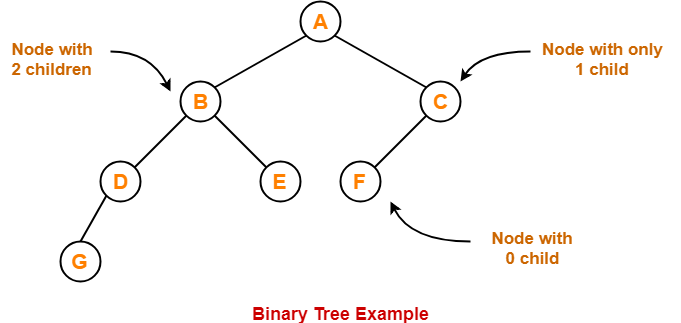## Unlabeled Binary Tree-

 A binary tree is unlabeled if its nodes are not assigned any label.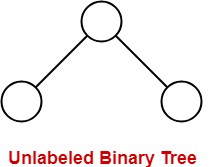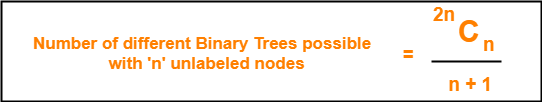## Example-

Consider we want to draw all the binary trees possible with 3 unlabeled nodes.

Using the above formula, we have-

Number of binary trees possible with 3 unlabeled nodes

2 x 3C3 / (3 + 1)

6C3 / 4

= 5

Thus,

• With 3 unlabeled nodes, 5 unlabeled binary trees are possible.
• These unlabeled binary trees are as follows-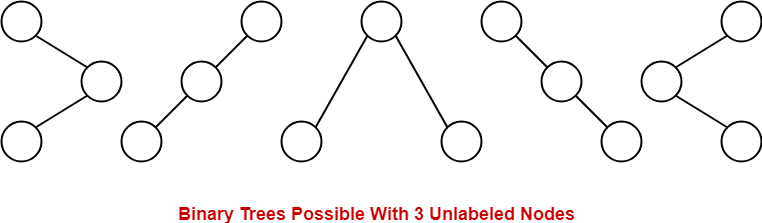## Labeled Binary Tree-

 A binary tree is labeled if all its nodes are assigned a label.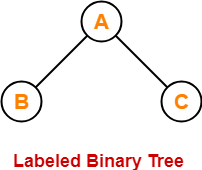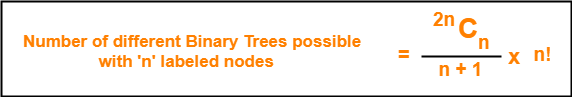## Example-

Consider we want to draw all the binary trees possible with 3 labeled nodes.

Using the above formula, we have-

Number of binary trees possible with 3 labeled nodes

= { 2 x 3C3 / (3 + 1) } x 3!

= { 6C3 / 4 } x 6

= 5 x 6

= 30

Thus,

• With 3 labeled nodes, 30 labeled binary trees are possible.
• Each unlabeled structure gives rise to 3! = 6 different labeled structures.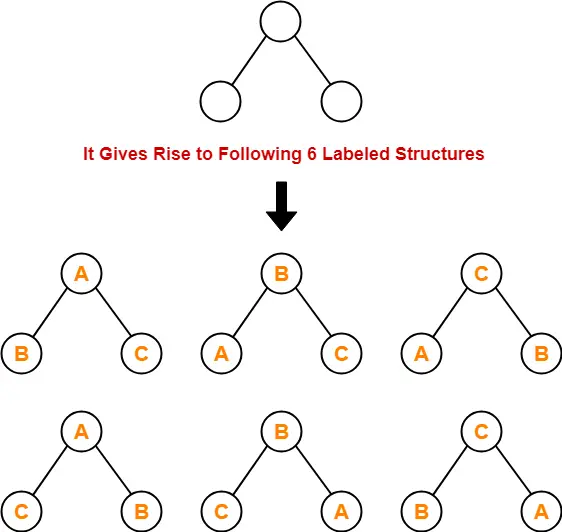Similarly,

• Every other unlabeled structure gives rise to 6 different labeled structures.
• Thus, in total 30 different labeled binary trees are possible.

## Types of Binary Trees-

Binary trees can be of the following types-1. Rooted Binary Tree
2. Full / Strictly Binary Tree
3. Complete / Perfect Binary Tree
4. Almost Complete Binary Tree
5. Skewed Binary Tree

## 1. Rooted Binary Tree-

A rooted binary tree is a binary tree that satisfies the following 2 properties-

• It has a root node.
• Each node has at most 2 children.

### Example-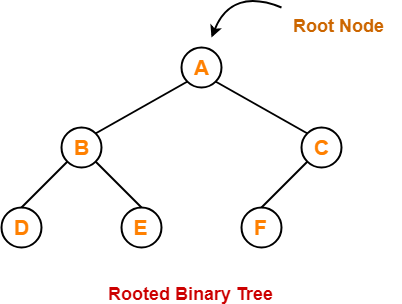## 2. Full / Strictly Binary Tree-

• A binary tree in which every node has either 0 or 2 children is called as a Full binary tree.
• Full binary tree is also called as Strictly binary tree.

### Example-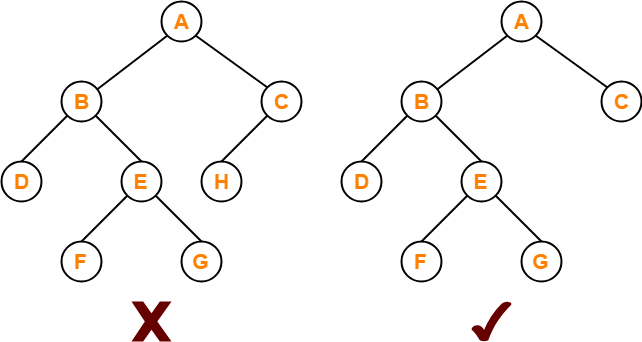Here,

• First binary tree is not a full binary tree.
• This is because node C has only 1 child.

## 3. Complete / Perfect Binary Tree-

A complete binary tree is a binary tree that satisfies the following 2 properties-

• Every internal node has exactly 2 children.
• All the leaf nodes are at the same level.

Complete binary tree is also called as Perfect binary tree.

### Example-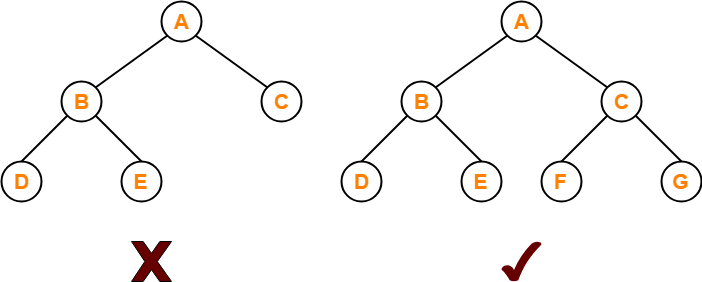Here,

• First binary tree is not a complete binary tree.
• This is because all the leaf nodes are not at the same level.

## 4. Almost Complete Binary Tree-

An almost complete binary tree is a binary tree that satisfies the following 2 properties-

• All the levels are completely filled except possibly the last level.
• The last level must be strictly filled from left to right.

### Example-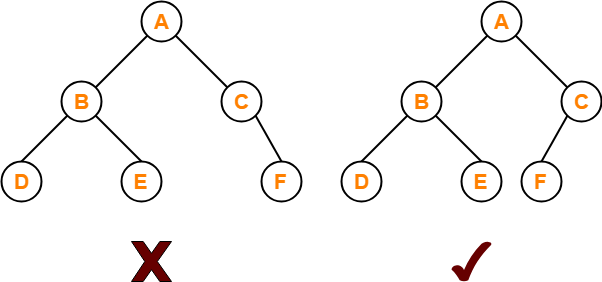Here,

• First binary tree is not an almost complete binary tree.
• This is because the last level is not filled from left to right.

## 5. Skewed Binary Tree-

skewed binary tree is a binary tree that satisfies the following 2 properties-

• All the nodes except one node has one and only one child.
• The remaining node has no child.

OR

A skewed binary tree is a binary tree of n nodes such that its depth is (n-1).

### Example-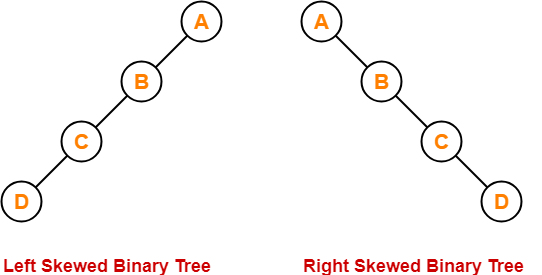To gain better understanding about Binary Tree and its types-

Watch this Video LectureNext Article- Binary Tree Properties

Get more notes and other study material of Data Structures.

Watch video lectures by visiting our YouTube channel LearnVidFun.